# SAT Math Multiple Choice Question 958: Answer and Explanation

### Test Information

Question: 958

10. A triangle is graphed on a standard coordinate plane. What is the perimeter of thetriangle if it has vertices (1, 4), (1, 7), and (4, 4)?

• A.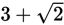• B.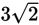• C.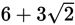• D.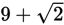Explanation:

C

Difficulty: Medium

Category: Additional Topics in Math / Geometry

Strategic Advice: Draw a quick sketch to help visualize the information. Don't spend a lot of time labeling hash marks-you just need a general idea of what the triangle looks like.

Getting to the Answer: The coordinates given in the question are plotted and connected in the figure below. We've labeled the vertices to aid with the explanation.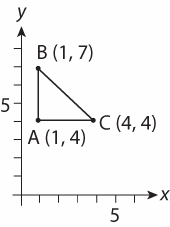Notice that one side of the triangle is parallel to the x-axis and another is parallel to the y-axis, so you can conclude that the legs are perpendicular, and the triangle formed is a right triangle. The leg lengths are easy to find-just subtract the appropriate coordinates to get lengths of 7 - 4 = 3 units and 4 - 1 = 3 units. This means the right triangle is isosceles and therefore a 45-45-90 triangle with legs of length 3. Thus, the hypotenuse measures. Add the three sides together to find the perimeter: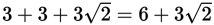, making (C) the correct answer.